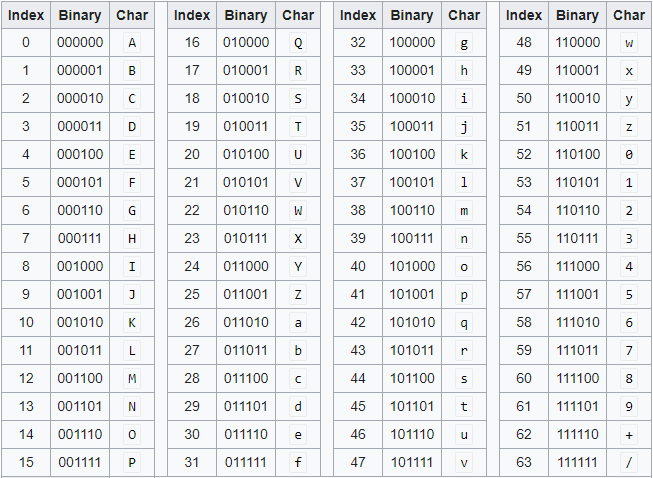GFG App
Open AppBrowser
Continue

# Encoding and Decoding Base64 Strings in Python

The Base64 encoding is used to convert bytes that have binary or text data into ASCII characters. Encoding prevents the data from getting corrupted when it is transferred or processed through a text-only system. In this article, we will discuss about Base64 encoding and decoding and its uses to encode and decode binary and text data.

Base64 encoding:
It is a type of conversion of bytes to ASCII characters. the list of available Base64 characters are mentioned below:

• 26 uppercase letters
• 26 lowercase letters
• 10 numbers
• + and / for new lines

Each Base64 character represents 6 bits of data. it is also important to note that it is not meant for encryption for obvious reasons.
To convert a string into a Base64 character the following steps should be followed:

• Get the ASCII value of each character in the string.
• Compute the 8-bit binary equivalent of the ASCII values
• Convert the 8-bit characters chunk into chunks of 6 bits by re-grouping the digits
• Convert the 6-bit binary groups to their respective decimal values.
• Use the Base64 encoding table to align the respective Base64 values for each decimal value.

The below image provides us with a Base64 encoding table.Image Source: Wikipedia

Using python to encode strings:
In Python the base64 module is used to encode and decode data. First, the strings are converted into byte-like objects and then encoded using the base64 module. The below example shows the implementation of encoding strings isn’t base64 characters.

Example:

 `import` `base64 ` ` `  `sample_string ``=` `"GeeksForGeeks is the best"` `sample_string_bytes ``=` `sample_string.encode(``"ascii"``) ` ` `  `base64_bytes ``=` `base64.b64encode(sample_string_bytes) ` `base64_string ``=` `base64_bytes.decode(``"ascii"``) ` ` `  `print``(f``"Encoded string: {base64_string}"``) `

Output:

`Encoded string: R2Vla3NGb3JHZWVrcyBpcyB0aGUgYmVzdA==`

Using Python to decode strings:
Decoding Base64 string is exactly opposite to that of encoding. First we convert the Base64 strings into unencoded data bytes followed by conversion into bytes-like object into a string. The below example depicts the decoding of the above example encode string output.

Example:

 `import` `base64 ` ` `  ` `  `base64_string ``=``" R2Vla3NGb3JHZWVrcyBpcyB0aGUgYmVzdA =="` `base64_bytes ``=` `base64_string.encode(``"ascii"``) ` ` `  `sample_string_bytes ``=` `base64.b64decode(base64_bytes) ` `sample_string ``=` `sample_string_bytes.decode(``"ascii"``) ` ` `  `print``(f``"Decoded string: {sample_string}"``) `

Output:

`Decoded string: GeeksForGeeks is the best`

My Personal Notes arrow_drop_up